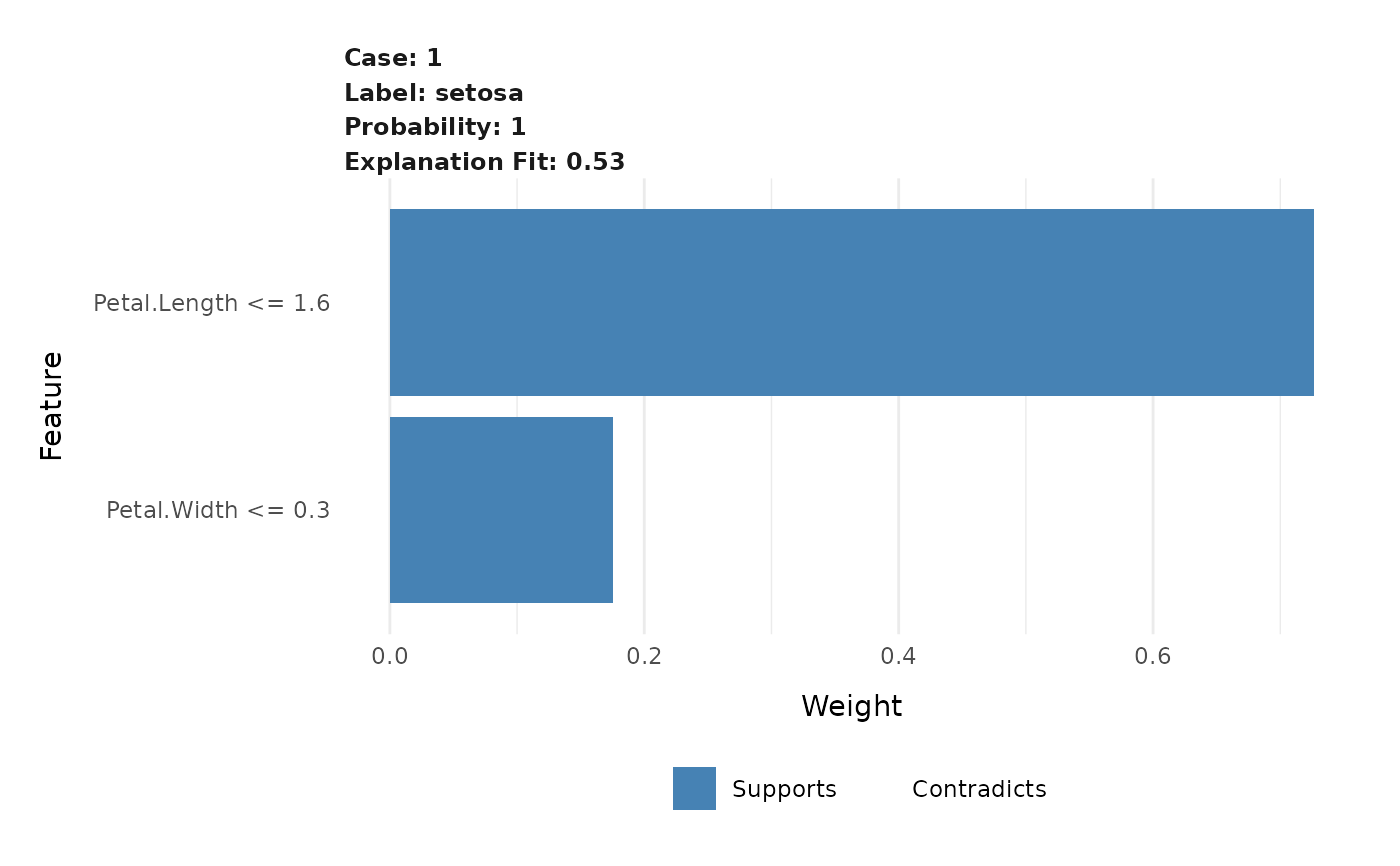This functions creates a compact visual representation of the explanations for each case and label combination in an explanation. Each extracted feature is shown with its weight, thus giving the importance of the feature in the label prediction.

plot_features(explanation, ncol = 2, cases = NULL)

## Arguments

explanation

A data.frame as returned by explain().

ncol

The number of columns in the facetted plot

cases

An optional vector with case names to plot. explanation will be filtered to only include these cases prior to plotting

## Value

A ggplot object

Other explanation plots: plot_explanations(), plot_text_explanations()

## Examples

# Create some explanations
library(MASS)
iris_test <- iris[1, 1:4]
iris_train <- iris[-1, 1:4]
iris_lab <- iris[][-1]
model <- lda(iris_train, iris_lab)
explanation <- lime(iris_train, model)
explanations <- explain(iris_test, explanation, n_labels = 1, n_features = 2)

# Get an overview with the standard plot
plot_features(explanations)